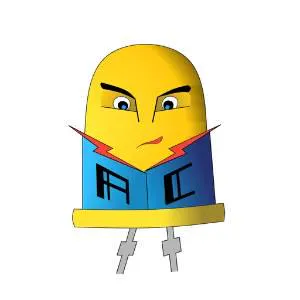# ArduBand - Save Your Eyes!

We could use a device reminding us of taking a short break to move and give a moment of respite to our eyes.

IntermediateFull instructions provided252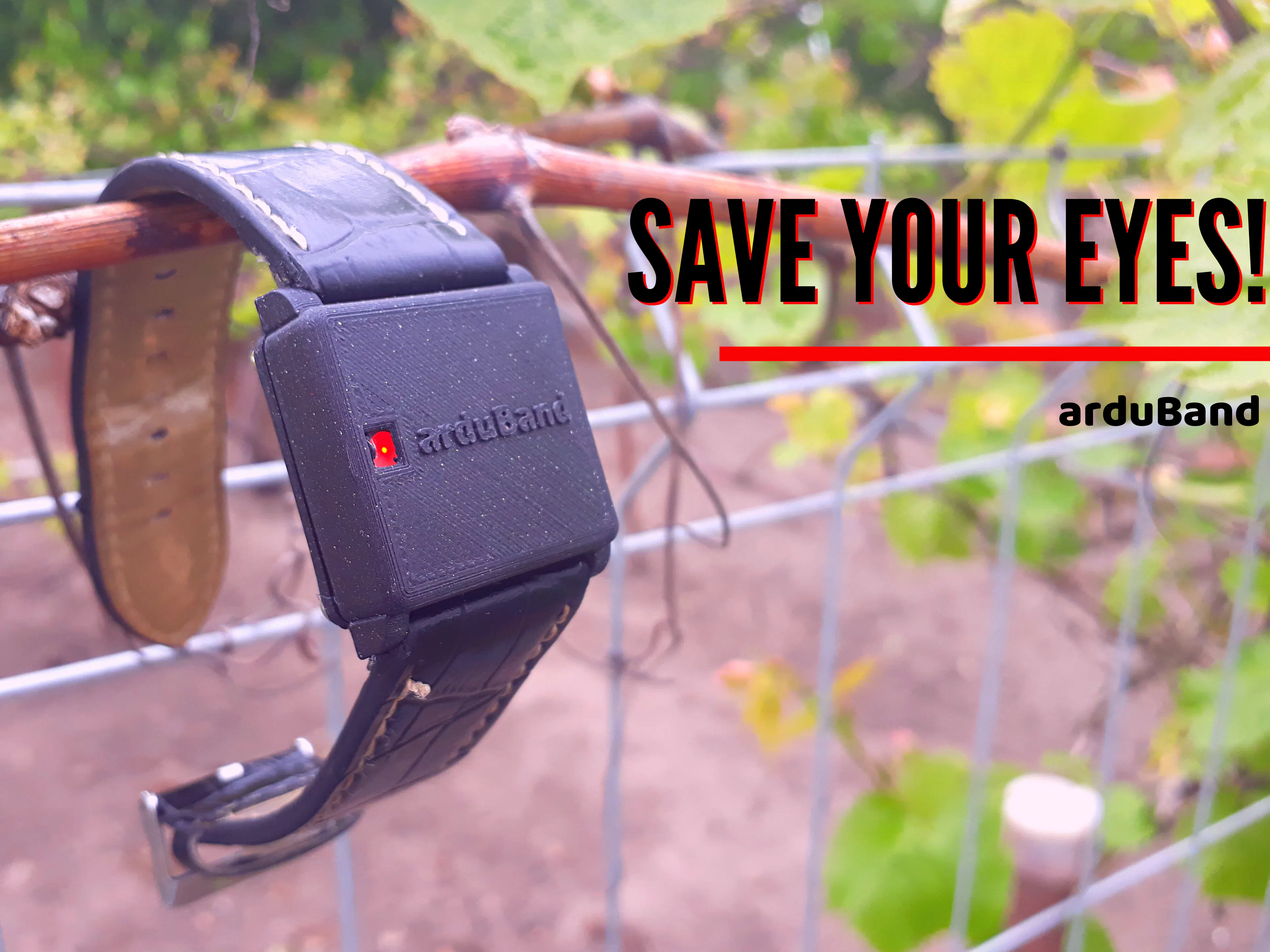## Things used in this project

### Hardware componentsArduino Nano R3
×1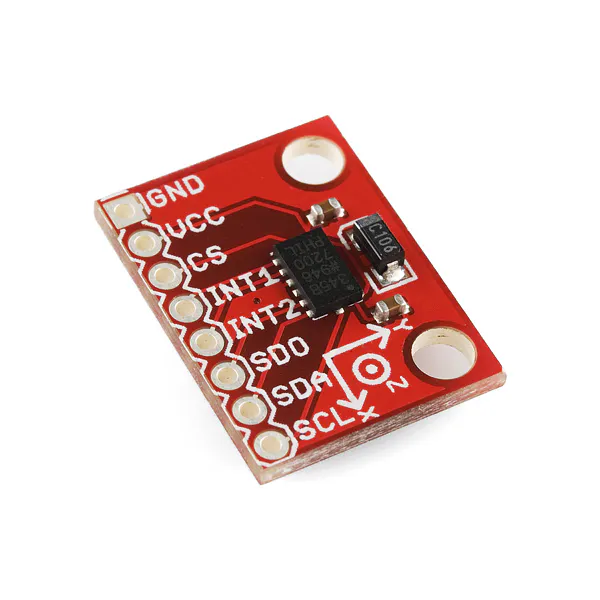SparkFun Triple Axis Accelerometer Breakout - ADXL345
×1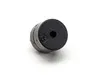Buzzer
×1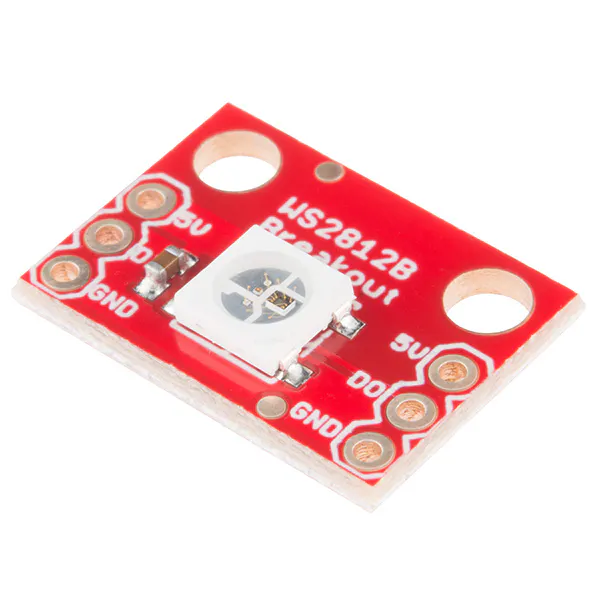SparkFun RGB LED Breakout - WS2812B
×1

### Software apps and online servicesArduino IDE

### Hand tools and fabrication machinesSoldering iron (generic)Solder Flux, Soldering3D Printer (generic)

Fusion 360 file

Schematic

PCB file

## Code

### arduBand code

C/C++
Code for arduBand
```#include <FastLED.h>

#include <Wire.h>
float X_out, Y_out, Z_out;
#include <avr/power.h>

#define DELAYVAL 500

#define PIN 10
#define NUMPIXELS 1
const byte motor = 11;
const byte piezo = 9;
unsigned long minutes;
unsigned long prevMinutes;
int myTime;
int myTime2;
int myPos;

int minMotorVal = 0;
int maxMotorVal = 50;

int a;
int i;

Adafruit_NeoPixel pixels(NUMPIXELS, PIN, NEO_GRB + NEO_KHZ800);

void setup() {
Serial.begin(9600);
pinMode(piezo, OUTPUT);
pinMode(motor, OUTPUT);
Wire.begin();
Wire.write(0x2D);
Wire.write(8);
Wire.endTransmission();
delay(10);
pixels.begin();
pixels.clear();
}
void loop() {
pixels.clear();
minutes = millis();
if (minutes - prevMinutes >= 1000)
{
prevMinutes = minutes;
myTime++;
}

if (myTime == 600)
{
checkValues();
checkPosition();

for (int i = 0; i <= 20; i++)
{
pixels.setPixelColor(0, pixels.Color(0, 0, i));
pixels.show();
delay(10);
}

for (int i = 20; i >= 0; i--)
{
pixels.setPixelColor(0, pixels.Color(0, 0, i));
pixels.show();
delay(10);
}
}

if (myTime == 1200)
{
checkValues();
checkPosition();

for (int i = 0; i <= 20; i++)
{
pixels.setPixelColor(0, pixels.Color(0, 0, i));
pixels.show();
delay(10);
}

for (int i = 20; i >= 0; i--)
{
pixels.setPixelColor(0, pixels.Color(0, 0, i));
pixels.show();
delay(10);
}
}

if (myTime >= 1800)
{
checkValues();
myPos++;
timeout();
}

Serial.print("myTime :");
Serial.println(myTime);
Serial.print("myTime2 :");
Serial.println(myTime2);
Serial.print("myPos :");
Serial.println(myPos);
delay(1000);
}

void checkValues()
{
Wire.write(0x32);
Wire.endTransmission(false);
X_out = X_out / 256;
Y_out = Y_out / 256;
Z_out = Z_out / 256;
}

void checkPosition()
{
if (Y_out <= 0.5)
{
myPos++;
}

if (Y_out > 0.5)
{
myPos = 0;
myTime = 0;
}
}

{
if (myPos >= 3)
{
analogWrite(motor, maxMotorVal);
delay(500);
analogWrite(motor, minMotorVal);
delay(500);
analogWrite(motor, maxMotorVal);
delay(500);
analogWrite(motor, minMotorVal);

for (int i = 0; i <= 20; i++)
{
pixels.setPixelColor(0, pixels.Color(i, 0, 0));
pixels.show();
delay(10);
}

for (int i = 20; i >= 0; i--)
{
pixels.setPixelColor(0, pixels.Color(i, 0, 0));
pixels.show();
delay(10);
}
}
}

void timeout()
{

if (Y_out < 0.5)
{
myTime2 = myTime;
}
if (Y_out >= 0.5)
{
myPos = 1;
if ((myTime2 + 60) == myTime)
{
tone(piezo, 1000);
delay(300);
tone(piezo, 1000);
delay(300);
tone(piezo, 2000);
delay(300);
noTone(piezo);
myPos = 0;
myTime = 0;
a = 0;
for (int i = 0; i <= 20; i++)
{
pixels.setPixelColor(0, pixels.Color(0, i, 0));
pixels.show();
delay(10);
}

for (int i = 20; i >= 0; i--)
{
pixels.setPixelColor(0, pixels.Color(0, i, 0));
pixels.show();
delay(10);
}
}
}
}
```

## Credits

### ardutronic

0 projects • 1 follower